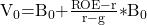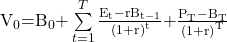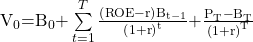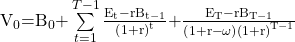101 Concepts for the Level I Exam

# Essential Concept 58: Residual Income ValuationIn a multistage residual income model, we assume different growth rates for the short term and the long-term.  We forecast the residual incomes over a short term horizon and estimate a terminal value based on the assumption made about continuing residual income over the long term.

Continuing residual income is residual income after the forecast horizon. Usually, one of the following assumptions is made for continuing residual income:

• Residual income continues indefinitely at a positive level.
• Residual income is zero from the terminal year forward.
• Residual income declines to zero as ROE reverts to the cost of equity through time.
• Residual income declines to some mean level.

If residual income continues indefinitely at a positive level, then we can use the following formula (single stage model):If at the end of time horizon T, a certain premium over book value (PT – BT ) exists for the company, then we can use the following formulas:If residual income fades over time, we can use the following formula:Where, ω = persistence factor between 0 and 1

1 ? residual income will not fade

0 ? residual income will not continue after the initial forecast horizon

The following table indicates characteristics associated with high and low levels of persistence:

 Lower residual income persistence Higher residual income persistence Extreme accounting rates of return (ROE) Low dividend payout Extreme levels of special items (e.g., nonrecurring items) High historical persistence in the industry Extreme levels of accounting accruals